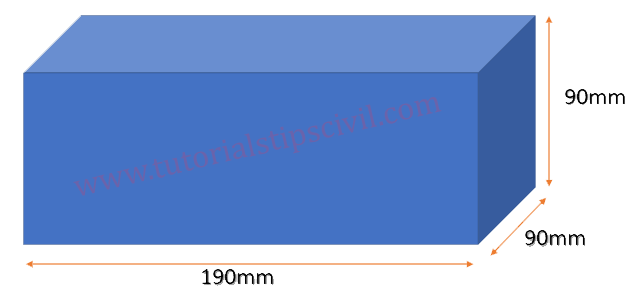How To Calculate Number Of Bricks, Cement And Sand For Brickwork?

Brickwork Calculation & Formula

Standard Size of Brick = 190 x 90 x 90 (mm)  Quality check of brick
Mortar Thickness is = 10 mm

Assume the size of brick work

Wall Size = 1 x 1 x 1 = 1 meter cube

First we are find volume of single brick without mortarVolume of Brick Without Mortar  = 0.19 x 0.09 x 0.09

= 0.001539 meter cube

Than we have to find volume of 1 brick with mortar

Volume of 1 Brick with Mortar = 0.20 x 0.1 x 0.1

= 0.002 meter cube

Number of brick required in brick work Volume = 1

Number of Bricks with mortar                     = 1/0.002    = 500 Nos.

Quantity of Mortar Volume is = Volume of wall – (Volume of Brick without mortar X Number of brick required )

Quantity of Mortar Volume is      = 1 –(0.001539 x 500)

= 1-0.7695 = 0.2305 meter cube

REQUIRED OF MORTAR IN BRICK WORK (Plaster Calculation)

Now we Calculate Mortar quantity of cement and sand Ratio is 1:6

Mortar quantity is 0.2305 (Wet Condition) we calculate Dry Value
Dry Mortar Value is = 0.2305 x 1.33 = 0.306565 m3

Cement Calculation

Volume = Volume of Mortar x (Ratio of Cement/ Sum of ratio)

Volume = 0.306565x (1/7) = 0.0438
Bags = 0.0438 x 1440 = 63.06 KG

Sand Calculation

Volume = Volume of Mortar x (Ratio of sand / Sum of ratio)

Volume = 0.306565x (6/7) = 0.2627
CFT = 0.2627 x 35.31 = 9.27841

Therefore- all materials required for 1 Cubic Meter Brick is,

Numbers of Brick- 500

Cement required – 63 kg

Sand Quantity     – 0.2627 Cubic Meter